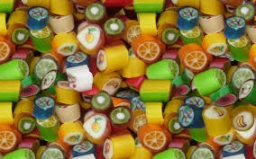# Kilograms 39911

In a luxury praline factory, they prepared 3.2 kg of a mixture of two types of candies for CZK 273 per 1 kg. Marzipan candies cost 345 CZK/kg and nougat 255 CZK/kg. How many kilograms of each type of candy were used?

a =  0.64
b =  2.56

### Step-by-step explanation:

a+b=3.2
273 · 3.2 = 345·a + 255·b

a+b = 3.2
345a+255b = 873.6

Pivot: Row 1 ↔ Row 2
345a+255b = 873.6
a+b = 3.2

Row 2 - 1/345 · Row 1 → Row 2
345a+255b = 873.6
0.26b = 0.67

b = 0.66782609/0.26086957 = 2.56
a = 873.6-255b/345 = 873.6-255 · 2.56/345 = 0.64

a = 16/25 = 0.64
b = 64/25 = 2.56

Our linear equations calculator calculates it.Did you find an error or inaccuracy? Feel free to write us. Thank you!

Tips for related online calculators
Do you have a linear equation or system of equations and looking for its solution? Or do you have a quadratic equation?